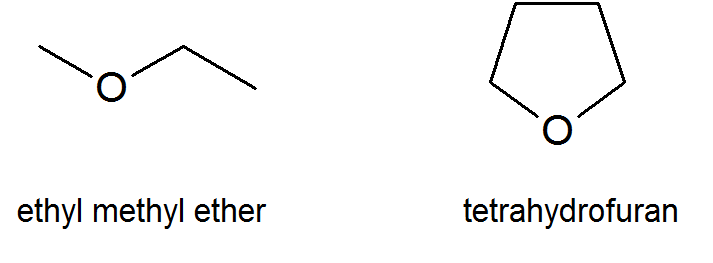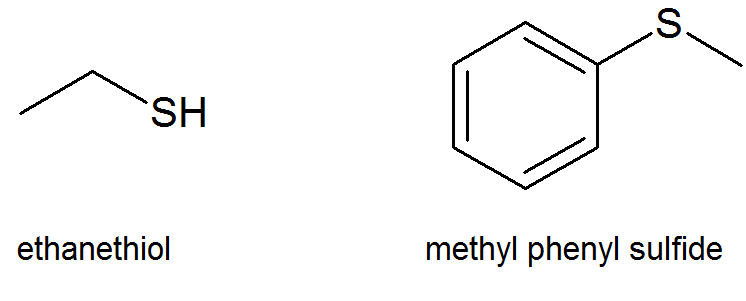# 18.0 Introduction

$$\newcommand{\vecs}{\overset { \rightharpoonup} {\mathbf{#1}} }$$ $$\newcommand{\vecd}{\overset{-\!-\!\rightharpoonup}{\vphantom{a}\smash {#1}}}$$$$\newcommand{\id}{\mathrm{id}}$$ $$\newcommand{\Span}{\mathrm{span}}$$ $$\newcommand{\kernel}{\mathrm{null}\,}$$ $$\newcommand{\range}{\mathrm{range}\,}$$ $$\newcommand{\RealPart}{\mathrm{Re}}$$ $$\newcommand{\ImaginaryPart}{\mathrm{Im}}$$ $$\newcommand{\Argument}{\mathrm{Arg}}$$ $$\newcommand{\norm}{\| #1 \|}$$ $$\newcommand{\inner}{\langle #1, #2 \rangle}$$ $$\newcommand{\Span}{\mathrm{span}}$$ $$\newcommand{\id}{\mathrm{id}}$$ $$\newcommand{\Span}{\mathrm{span}}$$ $$\newcommand{\kernel}{\mathrm{null}\,}$$ $$\newcommand{\range}{\mathrm{range}\,}$$ $$\newcommand{\RealPart}{\mathrm{Re}}$$ $$\newcommand{\ImaginaryPart}{\mathrm{Im}}$$ $$\newcommand{\Argument}{\mathrm{Arg}}$$ $$\newcommand{\norm}{\| #1 \|}$$ $$\newcommand{\inner}{\langle #1, #2 \rangle}$$ $$\newcommand{\Span}{\mathrm{span}}$$$$\newcommand{\AA}{\unicode[.8,0]{x212B}}$$

Objectives

After completing this section, you should be able to use the terms “ether,” “diethyl ether ” and “ethyl ether ” appropriately in context.

##### Key Terms

Make certain that you can define, and use in context, the key terms below.

• ether (R$\ce{-}$O$\ce{-}$R′)
• sulfide (R$\ce{-}$S$\ce{-}$R′)
• thiol (R$\ce{-}$S$\ce{-}$H)
##### Study Notes

As defined in the textbook, an “ether” is a substance with the general formula (R$\ce{-}$O$\ce{-}$R′) where R and R′are alkyl, aryl, vinyl or allyl groups. However, the word “ether” is also commonly used to refer to the specific compound, CH3$\ce{-}$CH2$\ce{-}$O$\ce{-}$CH2$\ce{-}$CH3, which is correctly called “diethyl ether.” Further confusion can arise because some chemists refer to “diethyl ether ” as “ethyl ether.” In this course, “ether ” will be used to refer to the class of compounds with the structure (R$\ce{-}$O$\ce{-}$R′); diethyl ether will refer to the compound, CH3$\ce{-}$CH2$\ce{-}$O$\ce{-}$CH2$\ce{-}$CH3; and “ethyl ether ” will not be used.

## Ethers and Epoxides

While the general formula for ethers is R-O-R′, keep in mind that there also cyclic ethers like tetrahydrofuran (a common organic solvent) or even epoxides which you first encounter in Section 8.7 in synthesizing diols from alkenes.## Thiols and Sulfides

Thiols (thio alcohols or mercaptans) and sulfides (thioethers) are the sulfur analogues of alcohols and ethers and have the general formulas of R-S-H and R-S-R′, respectively.